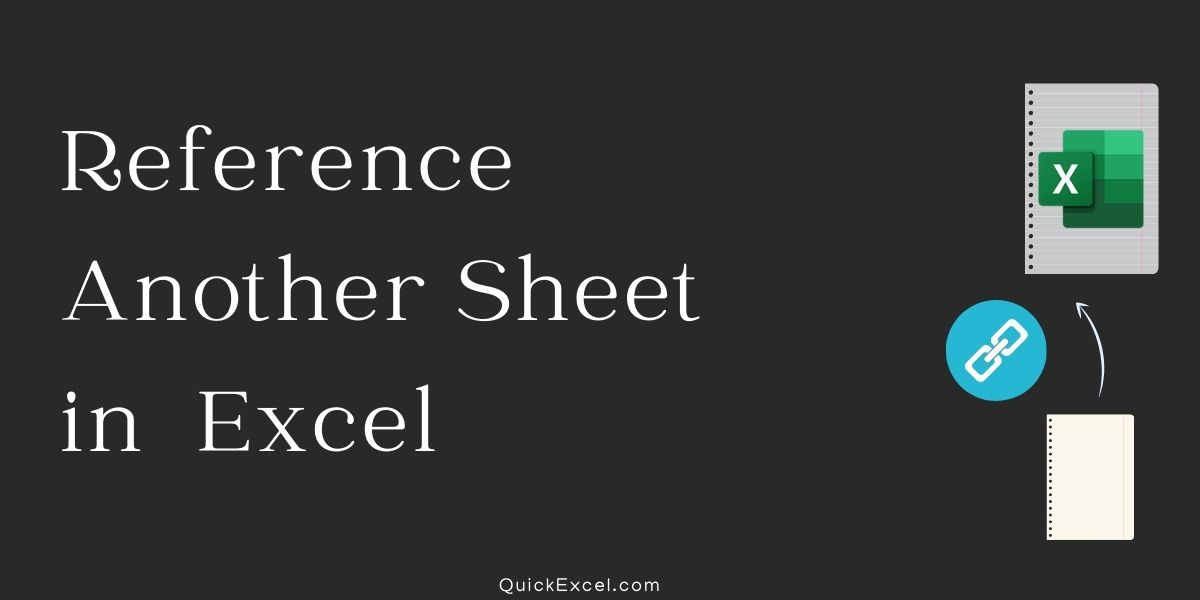# How to Reference Another Sheet in Excel?In this tutorial, we will explain to you the fundamentals of an external reference in Excel and demonstrate how you can reference another sheet and workbook in your formulas.

In Excel, an external reference refers to a cell or range of cells, not in the current worksheet. The significant advantage of utilizing an Excel external reference is that anytime the value supplied by the outer cell reference changes in another worksheet, the value returned by the external cell reference is instantly updated.

Whereas external references and cell references are pretty similar in Excel, there are a few key distinctions. In this lesson, we’ll start with the fundamentals and work our way up to creating various external references.

## 1. How to reference another worksheet

• Choose the cell where you want to enter the reference and click OK.
• To begin forming the reference, enter =.
• Choose the worksheet containing the cell you wish to refer to.
• Choose the cell you want to refer to.
• Enter by clicking or pressing the Enter key.
• Worksheets from Other Sources
• In the chosen cell, the value from the other worksheet displays.

NOTE: worksheet reference in a formula is denoted by an exclamation mark (!) following the sheet name.

## 2. How to Reference Another Excel file

Now you know how you can reference another worksheet. Now let us know how we can reference it from a completely different file.

• Select the location where you wish to place the reference.
• Before you can input the formula, you must first open the worksheet containing the data you want to reference.
• Enter = to begin constructing the connection.
• In the taskbar, choose the Excel icon.
• Choose the worksheet containing the cell(s) you wish to reference.
• Worksheets from Other Sources
• Choose the cell(s) to which you want to refer.
• Enter by clicking or pressing the Enter key.
• Worksheets from Other Sources

NOTE: Your formula will be broken if you move, delete, or rename the referenced file.

In the worksheet, the value from the other workbook shows.

When another file is mentioned in a formula, the file name is included in brackets, as seen below: [ ].

## 3. Reference in the same workbook but from a different sheet

Referencing a cell from the same sheet in Excel does not require rocket scientific expertise, and similarly, we may reference from separate worksheets in the same workbook.

Assume you have two sheets with the designations Sheet1 and Sheet2. We have data in Dataset(Sheet1), and we require the sum of these values in Sum(Sheet2).

1. Now, in Sheet2 and A2, open the SUM function.
2. Now, navigate to Sheet1 and pick the necessary cell range.
3. Close the formula by pressing the enter key.
4. Examine the formula reference =SUM in the Excel sheet now.

So, to refer to an excel cell or range of cells from another sheet, we must first obtain the worksheet name, i.e., Sheet1, and then an exclamation mark (!) before mentioning the cell location.

For a single-cell worksheet, name and cell address, for example, =Sheet1! B2

In this manner, we may refer to the various worksheet cells inside the same workbook. When we reference a cell from multiple worksheets in the same workbook, we get the sheet names before the cell reference.

Points to remember

1. We only obtain cell addresses when we reference cells from the same sheet.
2. When we reference a cell from another sheet in Excel that is part of the same workbook, we obtain the worksheet names and the cell location in that worksheet.
3. If we refer to the cell from another excel worksheet in the same workbook, we obtain a relative excel reference, i.e., A2.
4. If the cell is referenced from another Excel worksheet, we receive an absolute reference, i.e., \$A\$2.

## Conclusion

Now you had learned how to use Excel to refer to a cell in another sheet. You now have firsthand knowledge of how easy it is to reference another sheet in Excel.